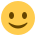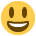#### Howdy, Stranger!

It looks like you're new here. If you want to get involved, click one of these buttons!

Supported by

# Bayesian one-sided independent samples t-test: question about the sequential analysis plot

Hi everyone
I am running a Bayesian independent samples t-test where I have specified that my hypothesis is that Group 2 has scored higher than Group 1. The sequential analysis plot looks as attached. Initially I thought the reason why the first ten participants do not move the evidence in either direction is because they are in Group 1, but Person 10 is in Group 2. Does this mean that the first 10 participants do not contribute to the evidence and only the information of the second group contributes? Is that a good thing or does this imply something may be wrong with my sample?

Kind regards
eniseg2

• Dear Eniseg2,

Nothing is wrong (although...see below). If you test whether two groups are different, and all you see are data from one of the groups, the BF should remain exactly 1. I am not so sure about the role of the 10th participant, however. It may be that we've hardcoded the BF to remain 1 until each group has had more than 1 participant. That does not seem exactly correct, however, because sigma is common to both groups and so the first observation from the second group should be informative. Maybe I am missing something. I'll forward this to the expert and get back to you.

Cheers,
E.J.

• edited August 2018

Hi Eniseg2,

Thanks for this report. There's nothing wrong with your data and your intuition is spot on. The first 9 Bayes factors are 1, and this is correct. The 11th Bayes factor and onwards are also correct. So there's no need to worry about those either. The 10th point Bayes factor, however, does not need to be 1.

A two-sample Bayesian t-test with a prior centred at zero is 1, whenever the data set is uninformative. For the two-sample comparison at hand, there are two (and only two) types of data sets that are uninformative, namely:

• i. Data sets with all observations in one group [no information about the mean difference to calculate a t-value, and the degrees of freedom is 0], or
• ii. Data sets consisting of only two observations with n1=1 and n2=1 [no information about the standard deviation].

These uninformative data sets are actually hard to handle on a computer, but doing the calculations by hands shows that the (i) and (ii) indeed leads to BF10=1 (whenever the prior is centred at zero). To avoid doing the expensive calculations, we should therefore just return one, whenever (i) or (ii) hold.

Since (i) and (ii) characterise all types of uninformative data sets for the Bayesian t-test, we conclude that this Bayes factor accumulates evidence whenever the following condition is met:

• (a) the total sample size is at least 3, thus, n >= 3, and each group has at least 1 observation. Thus, n>=3 and n1 >= 1 or n2 >= 1.

In the code, the or was implemented as an and resulting in:

• (b) n1 >=2 and n2 >= 2 [which automatically implies n >= 4].

Note that (b) implies (a). The case with n1=9 and n2=1 is exactly at the boundary and fulfils (a), but not (b). So a Bayes factor should be calculated, but the code doesn't do so. For all subsequent data points, thus, the 11th Bayes factor and onwards, condition (b) and therefore (a) is met, so those are correct.

We're currently revising our code, so I'll take this into account. Thanks for the find. I hope that this answers your question. For the mathematical details behind EJ's explanation, please see Section 2.3.1 of (https://arxiv.org/abs/1704.02479v2).

Cheers,
Alexander

• Hi EJ and Alexander,
sorry for my delayed reaction to this and thank you so much for your kind help. It took me two or three reads to fully get the picture but the little lightbulb in my head just came on.I just wanted to say how much I appreciate all the help I've been getting in this forum, and how fantastic JASP is. I used JASP to teach my postgraduate students earlier this year. One of them is currently on placement and someone at the host institution asked my student to help them convince the institution to buy SPSS instead of using JASP. My student told them that she'd been trained in JASP and thought it was much better than SPSS so she wouldn't support paying for SPSS! I was a little proud.Thank you so much!
Anna

• :-)

• Hi Anna,

Thanks for your encouraging words. Can you please let me know which part of my comment was particular hard to parse? Then I'll be able to explain it better next it comes up.

Thanks,
Alexander